SymmetryWorks Mini-Tutorials

# Determining the Type of Symmetry of a Repeating Pattern

How do you find the symmetry type of a pattern? Easy: by answering at most four questions, starting with this one: Will the pattern coincide with itself if it is rotated around some center?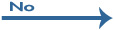Is there a reflection?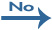Is there a glide-reflection?Simple shift (p1)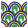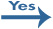Glide reflection (pg)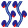Is there a glide-reflection in an axis that is not a reflection axis?Mirror (pm)Mirror & glide (cm)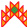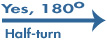Is there a reflection?Is there a glide-reflection?Half-turn (p2)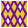Double glide (pgg)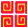Are there reflections in two directions?Parallel mirrors & glide (pmg)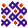Are all rotation centers on reflection axes?Perpendicular mirrors & glide (cmm)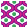Double mirror (pmm)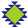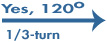Is there a reflection?Three rotations (p3)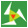Are there reflections in two directions?Three rotations & mirrors (p31m)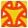Three mirrors (p3m1)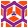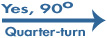Is there a reflection?Pinwheel (p4)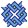Are there four reflection axes?Quarter-turns & rotated mirrors (p4g)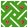Quarter-turns & mirrors (p4m)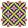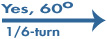Is there a reflection?Six rotations (p6)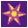Kaleidoscope (p6m)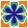Adopted from McLenaghan and Levy, 1996, p. 264.